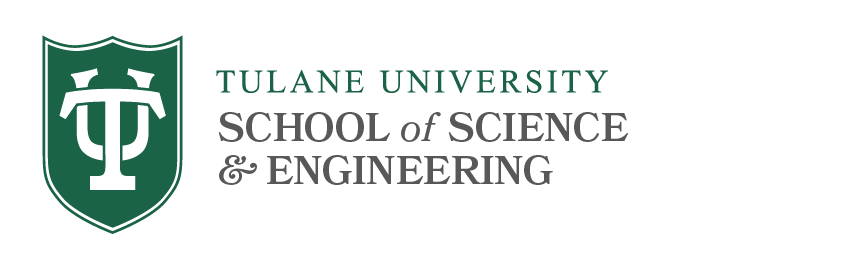# Topology Qualifying Exams

## Syllabus Topics

This exam covers three basic areas of topology:

1. Basic point set topology
2. Fundamental group and covering spaces
3. Algebraic topology-homology theory

On the exam you will be expected to:

• state definitions and major theorems
• give complete proofs for more elementary results on the spot
• sketch the main outline for proofs of major results
• be able to prove simple propositions utilizing key definitions
• be able to apply main theorems to prove other results (e.g. typical homework problem with one page proof)
• follow outlines to prove multistep results
• know certain basic facts (π ₁ (S¹ ), homology of spheres and (disk, sphere) pairs)
• use principles such as homotopy invariance, excision, Mayer-Vietoris, cellular or simplicial homology to do computations

One is not expected to memorize proofs of major theorems such as the homotopy invariance of homology-the emphasis is on knowing the statements and being able to apply the results. However, in some cases, you will be expected to be able to sketch the proof of the theorem.

Below we will distinguish theorems by SSA for "state, sketch the proof, and apply" and SA for "state and apply." This listing of theorems is not intended to be complete but we do try to mention the most important ones.

For each of the 3 areas, there is a primary reference. If you know the material from this primary reference, this is sufficient. We will also include some secondary references for further research or additional viewpoints.

Looking at the last five written examinations should give a good sense of the range of problems asked.

### 1. Basic Point Set Topology

• General definitions of topological spaces: open and closed sets, bases and sub-bases for topology, subspaces and subspace topology, continuous functions, homeomorphism.
• Metric spaces: bases and sub-bases for topology, equivalent metrics, Cauchy sequences and completeness, Baire category Theorem (SSA).
• Separation properties: Hausdorff and normal spaces, Urysohn's lemma (SSA) and Tietze extension theorem (SSA), relation to metric spaces.
• Compactness: basic definitions, properties: e.g. closed subset of compact is compact,compact Hausdorff is normal, continuous image of compact is compact (should be able to prove and use all of these), Compactness for metric spaces: compactness, sequential compactness, complete and totally bounded sets (SSA), Heine-Borel Theorem (SSA), second countability and Lindelof's Theorem (SSA).
• Local compactness, one-point compactification.
• Connectedness, components, relations to path connectedness (implications and counterexamples), local connectedness and local path connectedness.
• Product spaces, Tychonoff's Theorem (SSA), connectedness and path connectedness.
• Infinite product spaces, Tychonoff's Theorem for infinite products (SSA), connectedness and path connectedness.
• Quotient spaces and quotient topology: definitions and use in examples such as showing a map from a quotient space is continuous, or there is a homeomorphism from the quotient to D ⁿ/S ⁿ⁻¹ to S ⁿ.

References

Primary Reference:
1. Gamelin and Greene, Introduction to Topology, Chs I,II.

Secondary References:
2. Munkres, Topology, A First Course, Chs 2-4,5.1,7.1,7.3,7.7.
3. Bredon, Topology and Geometry, Ch 1.

### 2. Fundamental Group and Covering Spaces

• Basic homotopies, path homotopies, relative endpoints
• Definition of fundamental group, group properties such as associativity, inverses
• Fundamental group of a circle, and related computations such as fundamental group of the punctured plane
• Covering spaces: basic definitions, examples
• Path lifting, relationship of fundamental group and covering spaces
• Universal covering spaces, classification of covering spaces in terms of subgroups of the fundamental group
• Computations of π using covering spaces, homotopy properties (e.g. a Möbius band deformation retracts to the center circle so π ₁ ≃ Ζ ).
• Applications of fundamental group: e.g. Brouwer fixed point theorem for D ² (SSA)

References

Primary Reference:
1. Gamelin and Greene, Introduction to Topology, Ch III, 1-8.

Secondary References:
2. Munkres, Topology, A First Course, Ch 8.
3. Bredon, Topology and Geometry, Ch III.
4. Massey, Algebraic Topology, an Introduction, Chs 2, 4, 5 (This book gives a more complete treatment of topics such as van Kampen's theorem and covering spaces than some of the other references.)

### 3. Algebraic Topology - Homology Theory

• Basic definitions and constructions of singular homology: singular chain complex, H ⁰ and path components.
• Basic homological algebra (Bredon IV.5): working with chain complexes, exact sequences, five lemma.
• Axioms (characterizing properties of singular homology for simplicial complexes): homotopy invariance (SA), exact homology sequence for pair (SA), excision (SA), dimension axiom (SA).
• Mayer-Vietoris sequence (SA): e.g. compute homology of Klein bottle by dividing it into two annuli.
• CW complexes, cellular homology, computations: e.g. compute homology of projective plane or surfaces from cellular chain complex, special case of simplicial complexes and simplicial homology.
• Applications: students are expected to know basic facts such as homology of spheres and to do computations using axioms, Mayer-Vietoris sequence or techniques such as cellular homology computations, as in computation for projective spaces. Specific applications include the Brower fixed point theorem (SSA), invariance of domain (SSA), Borsuk-Ulam Theorem (SSA), Ham Sandwich Theorem (SSA), Generalized Jordan Curve Theorem (SSA), Lefschetz-Hopf Fixed Point Theorem (SSA).

References

Primary Reference:
1. Bredon, Topology and Geometry, Ch 4.

Secondary References:
2. Munkres, Elements of Algebraic Topology, Chs 1-4.
3. Greenberg and Harper, Algebraic Topology, Part II.